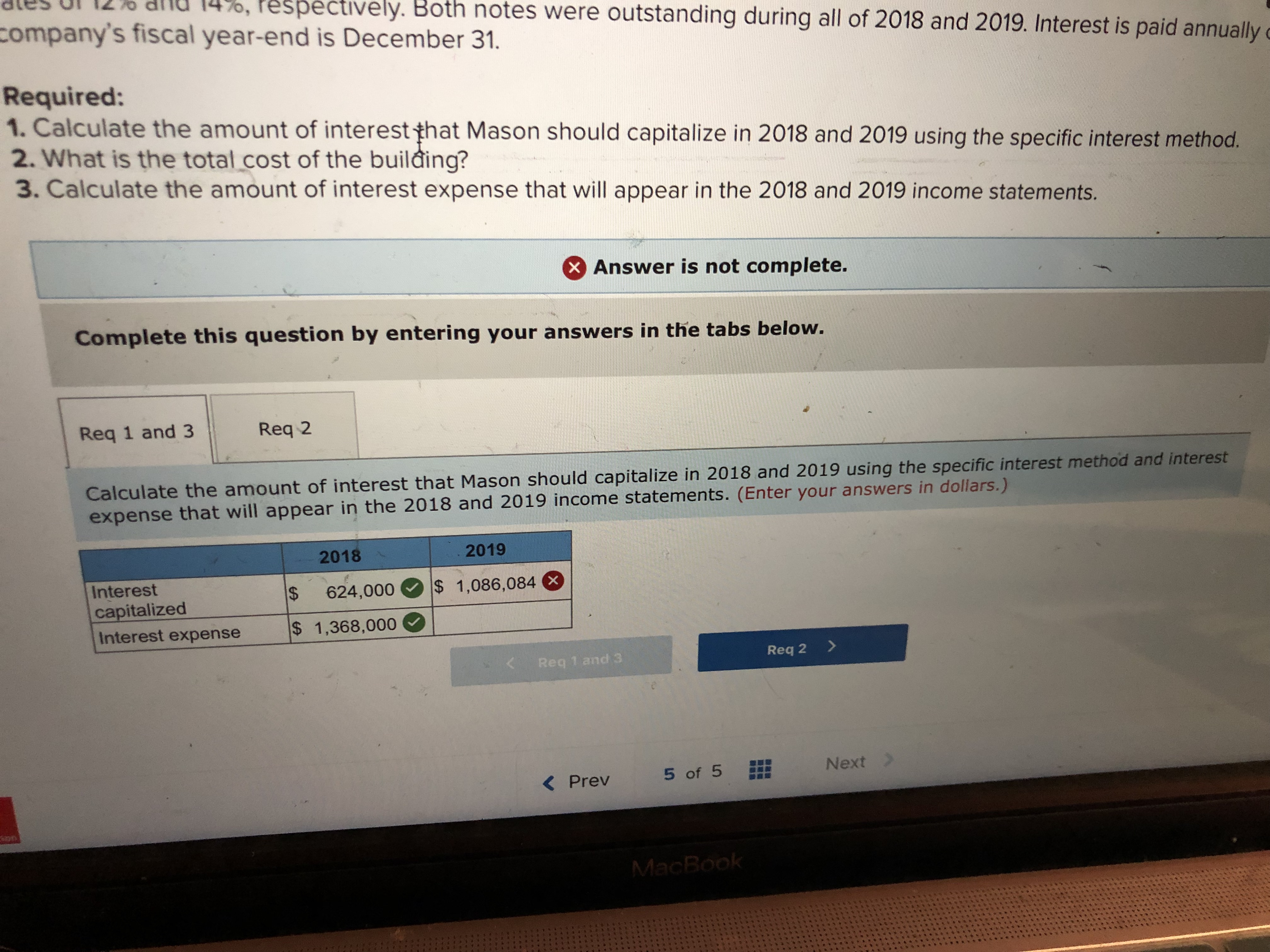pectively. Both notes were outstanding during all of 2018 and 2019. Interest is paid annuallycompany's fiscal year-end is December 31.Required:1. Calculate the amount of interest that Mason should capitalize in 2018 and 2019 using the specific interest method.2. What is the total.cost of the building?3. Calculate the amount of interest expense that will appear in the 2018 and 2019 income statements.Answer is not complete.Complete this question by entering your answers in the tabs below.Req 1 and 3 Req 2Calculate the amount of interest that Mason should capitalize in 2018 and 2019 using the specific interest method and interestexpense that will appear in the 2018 and 2019 income statements. (Enter your answers in dollars.)20182019InterestcapitalizedInterest expense s 1,368,000624,00o 1,086,084Req 1 and 3Req 2>< Prev 5of 5 Next

Question

On January 1, 2018, the Mason Manufacturing Company began construction of a building to be used as its office headquarters. The building was completed on September 30, 2019.

Expenditures on the project were as follows:

 January 1, 2018 \$ 1,710,000 March 1, 2018 1,320,000 June 30, 2018 1,520,000 October 1, 2018 1,320,000 January 31, 2019 378,000 April 30, 2019 711,000 August 31, 2019 1,008,000

On January 1, 2018, the company obtained a \$4,200,000 construction loan with a 16% interest rate. The loan was outstanding all of 2018 and 2019. The company’s other interest-bearing debt included two long-term notes of \$4,000,000 and \$6,000,000 with interest rates of 12% and 14%, respectively. Both notes were outstanding during all of 2018 and 2019. Interest is paid annually on all debt. The company’s fiscal year-end is December 31.

Required:
1. Calculate the amount of interest that Mason should capitalize in 2018 and 2019 using the specific interest method.
2. What is the total cost of the building?
3. Calculate the amount of interest expense that will appear in the 2018 and 2019 income statements.

I keep getting the wrong answer for total capitalization and expense

also i try doing the cost of the buildinghelp_outlineImage Transcriptionclosepectively. Both notes were outstanding during all of 2018 and 2019. Interest is paid annually company's fiscal year-end is December 31. Required: 1. Calculate the amount of interest that Mason should capitalize in 2018 and 2019 using the specific interest method. 2. What is the total.cost of the building? 3. Calculate the amount of interest expense that will appear in the 2018 and 2019 income statements. Answer is not complete. Complete this question by entering your answers in the tabs below. Req 1 and 3 Req 2 Calculate the amount of interest that Mason should capitalize in 2018 and 2019 using the specific interest method and interest expense that will appear in the 2018 and 2019 income statements. (Enter your answers in dollars.) 2018 2019 Interest capitalized Interest expense s 1,368,000 624,00o 1,086,084 Req 1 and 3 Req 2> < Prev 5of 5 Next fullscreen
Step 1

Capitalized Interest:

Capitalized Interest refers to the interest charge on the acquisition of long term loans or assets. It is not an interest expense which is shown in the income statement rather it is capitalized and depreciated over the years.

Step 2

1.

2018

Calculation of total expenditure:

Calculation of capitalized interest:

Thus, the Capitalized Interest for 2018 is \$624,000.

Step 3

2019

Project completed on September 30, 2019, so interest expense will be for 9 months for 2019, which is from January to September.

Calculation of total expenditure:

Thus, the to...

Want to see the full answer?

See Solution

Want to see this answer and more?

Our solutions are written by experts, many with advanced degrees, and available 24/7

See Solution
Tagged in